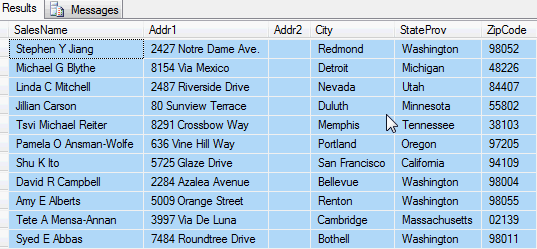# SQL Server Parsing

This tutorial examines techniques to parse out the City, State and Zip Code of Addresses in a table in an Address1 through 3 format.

## SQL Server Mailing Address Issue Background

Data is not always provided in a format that can be readily imported or used. For example, the following table constructed in our AdventureWorks database has the following structure of Name and Address Lines 1 -3.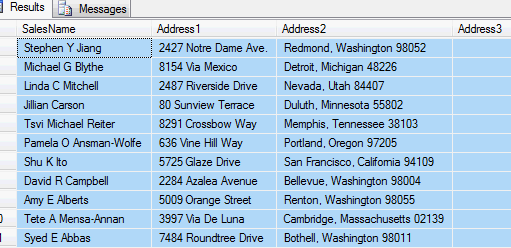If we needed to separate the City, State and Zip of these addresses, we could do so by adding a few fields and creating a stored procedure.

To add the fields, we can open a Query window in SSMS and use the following ALTER TABLE syntax to add fields to capture the last line in the address and the City, State and Zip.

City nvarchar(150) null,
StateProv nvarchar(100) null,
ZipCode nvarchar(20) null

## Code the SQL Stored Procedure

With these fields added, we can construct a stored procedure to:
1. Extract the last line of the address into the CSZ field
2. Use the SUBSTRING and REPLACE functions of T-SQL to parse out the ZipCode, then the City and State

AS
--search for and set the CSZ (CityStateZip) field to the last line in the address

--Get the separate City, State and Zip fields

ltrim(substring (CSZ, len(CSZ)+1- charindex(' ' ,reverse(replace(CSZ,'-',''))), len(CSZ)))
where isnumeric (substring (reverse(replace(CSZ,'-','')),1,4)) = 1

/*to set the City field use SUBSTRING on the CSZ field position 1 to start and
CHARINDEX to indicate the end position.
*to set the StateProv field, use REPLACE on the SUBSTRING of CSZ. Start position is LENGTH of the SUBSTRING of the CSZ from position 1 to the CHARINDEX of the comma + 1. End position is the LENGTH of the CSZ. To get the Zip and set it to '' use a 2nd SUBSTRING of the CSZ from the space between the State and Zip by looking from the end of CSZ backwards w/REVERSE */

UPDATE tblSalesAddresses SET City= case when charindex(',',CSZ)> 0 then
substring(CSZ, 1, convert(integer, charindex(',',CSZ))-1) end,
stateProv = case when charindex(',',CSZ)> 0 then
ltrim(replace(substring(CSZ, len(substring(CSZ, 1, convert(integer, charindex(',',CSZ))))+1, len(CSZ)), ltrim(substring (CSZ, len(CSZ)+1-convert (int,charindex(' ',reverse(replace(CSZ,'-','')))), len(CSZ))),''))end
where CSZ is not null

UPDATE tblSalesAddresses SET ZipCode = substring(ZipCode,1,5)

## Test the SQL Address Parsing Code

The first UPDATE statements simply look at the table for the last non null field populated.
The ZipCode Update uses the SUBSTRING, CHARINDEX, REVERSE, REPLACE, ISNUMERIC functions to extract the ZipCode from the end of the CSZ field. Here is what the data looks like when using a SELECT statement.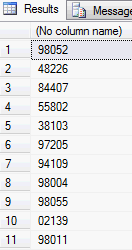select ltrim(substring (CSZ, len(CSZ)+1- charindex(' ', reverse(replace(CSZ,'-',''))), len(CSZ)))
where countryRegionName like 'united states'

The UPDATE statement to extract the City uses the SUBSTRING function on the CSZ field from position 1 to the CHARINDEX position of the comma separating the City and State.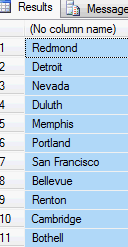select case when charindex(',',CSZ)> 0 then
substring(CSZ, 1, convert(integer, charindex(',',CSZ))-1) end
where countryRegionName like 'united states'

The UPDATE statement to REPLACE the StateProv + Zip from the CSZ field uses the SUBSTRING from the position of the comma +1 to the end of the CSZ. It then uses the SUBSTRING from the (CSZ end position - the position of the space between the State and Zip) to the end of the CSZ field as the String to REPLACE in our first SUBSTRING of StateProv + Zip.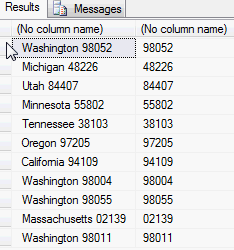select substring(CSZ, len(substring(CSZ, 1, convert(integer, charindex(',',CSZ))))+1, len(CSZ)),
ltrim(substring (CSZ, len(CSZ)+1-
convert (int,charindex(' ',reverse(replace(CSZ,'-','')))),
len(CSZ)))
where countryRegionName like 'united states'